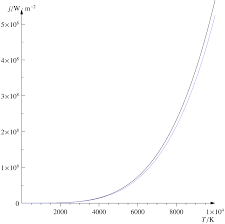## How to Calculate and Solve for Stefan Boltzmann’s Constant | Radiation Heat TransferThe image above represents stefan boltzmann’s constant.

To compute for stefan boltzmann’s constant, three essential parameters are needed and these parameters are Boltzmann’s Constant (KB), Planck’s Constant (h) and Speed of Light (c).

The formula for calculating stefan boltzmann’s constant:

σ = 2π5KB4 / 15c²h5

Where:

σ = Stefan Boltzmann’s Constant
KB = Boltzmann’s Constant
h = Planck’s Constant
c = Speed of Light

Let’s solve an example;
Find the stefan boltzmann’s constant when the boltzmann’s constant is 42, the planck’s constant is 34 and the speed of light is 26.

This implies that;

KB = Boltzmann’s Constant = 42
h = Planck’s Constant = 34
c = Speed of Light = 26

σ = 2π5KB4 / 15c²h5
σ = 2π5(42)4 / 15(26)²(34)5
σ = 2(306.019)(3111696) / 15(676)(45435424)
σ = 1904480458.135 / 460715199360
σ = 0.00413

Therefore, the stefan boltzmann’s constant is 0.00413.